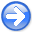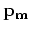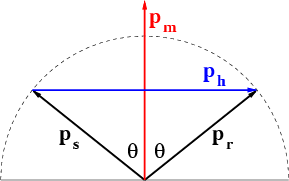Time-shift imaging condition in seismic migrationNext: Space-shift imaging condition Up: Time-shift imaging condition in Previous: Space-shift and time-shift imaging

# Angle transformation in wave-equation imaging

Using the definitions introduced in the preceding section, we can make the standard notations for source and receiver coordinates:and. The traveltime from a source to a receiver is a function of all spatial coordinates of the seismic experiment. Differentiatingwith respect to all components of the vectorsand, and using the standard notations, where, we can write:(11)(12)

From equations (11)-(12), we can write(13)(14)vec3
Figure 1.
Geometric relations between ray vectors at a reflection point.By analyzing the geometric relations of various vectors at an image point (Figure 1), we can write the following trigonometric expressions:(15)(16)

Equations (15)-(16) relate wavefield quantities,and, to a geometric quantity, reflection angle. Analysis of these expressions provide sufficient information for complete decompositions of migrated images in components for different reflection angles.

SubsectionsTime-shift imaging condition in seismic migrationNext: Space-shift imaging condition Up: Time-shift imaging condition in Previous: Space-shift and time-shift imaging

2007-04-08